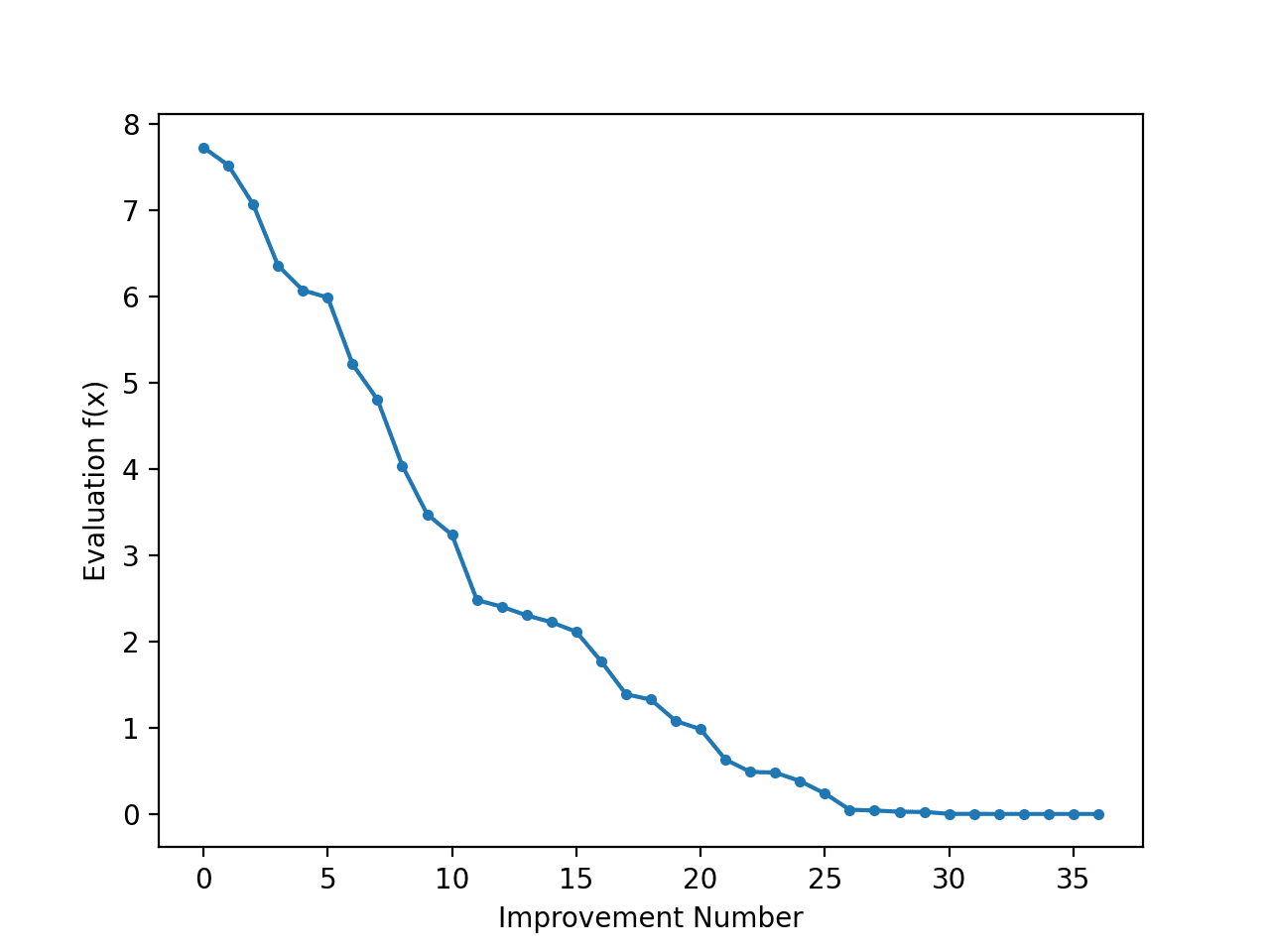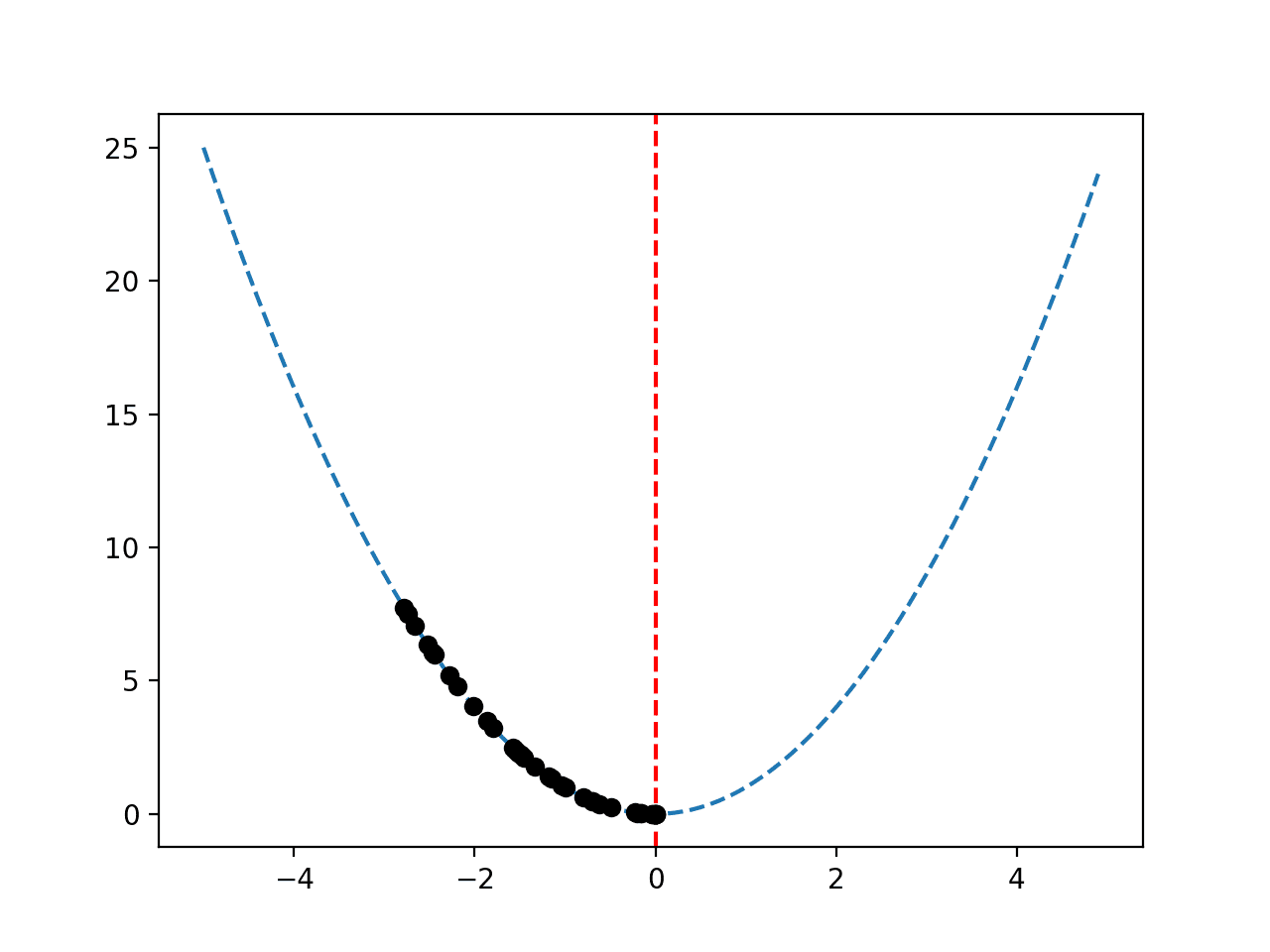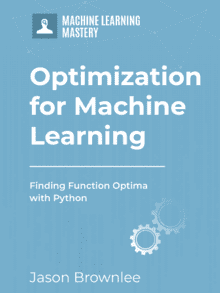# Stochastic Hill Climbing in Python from Scratch

Last Updated on October 12, 2021

Stochastic Hill climbing is an optimization algorithm.

It makes use of randomness as part of the search process. This makes the algorithm appropriate for nonlinear objective functions where other local search algorithms do not operate well.

It is also a local search algorithm, meaning that it modifies a single solution and searches the relatively local area of the search space until the local optima is located. This means that it is appropriate on unimodal optimization problems or for use after the application of a global optimization algorithm.

In this tutorial, you will discover the hill climbing optimization algorithm for function optimization

After completing this tutorial, you will know:

• Hill climbing is a stochastic local search algorithm for function optimization.
• How to implement the hill climbing algorithm from scratch in Python.
• How to apply the hill climbing algorithm and inspect the results of the algorithm.

Kick-start your project with my new book Optimization for Machine Learning, including step-by-step tutorials and the Python source code files for all examples.

Let’s get started.Stochastic Hill Climbing in Python from Scratch
Photo by John, some rights reserved.

## Tutorial Overview

This tutorial is divided into three parts; they are:

1. Hill Climbing Algorithm
2. Hill Climbing Algorithm Implementation
3. Example of Applying the Hill Climbing Algorithm

## Hill Climbing Algorithm

The stochastic hill climbing algorithm is a stochastic local search optimization algorithm.

It takes an initial point as input and a step size, where the step size is a distance within the search space.

The algorithm takes the initial point as the current best candidate solution and generates a new point within the step size distance of the provided point. The generated point is evaluated, and if it is equal or better than the current point, it is taken as the current point.

The generation of the new point uses randomness, often referred to as Stochastic Hill Climbing. This means that the algorithm can skip over bumpy, noisy, discontinuous, or deceptive regions of the response surface as part of the search.

Stochastic hill climbing chooses at random from among the uphill moves; the probability of selection can vary with the steepness of the uphill move.

— Page 124, Artificial Intelligence: A Modern Approach, 2009.

It is important that different points with equal evaluation are accepted as it allows the algorithm to continue to explore the search space, such as across flat regions of the response surface. It may also be helpful to put a limit on these so-called “sideways” moves to avoid an infinite loop.

If we always allow sideways moves when there are no uphill moves, an infinite loop will occur whenever the algorithm reaches a flat local maximum that is not a shoulder. One common solution is to put a limit on the number of consecutive sideways moves allowed. For example, we could allow up to, say, 100 consecutive sideways moves

— Page 123, Artificial Intelligence: A Modern Approach, 2009.

This process continues until a stop condition is met, such as a maximum number of function evaluations or no improvement within a given number of function evaluations.

The algorithm takes its name from the fact that it will (stochastically) climb the hill of the response surface to the local optima. This does not mean it can only be used for maximizing objective functions; it is just a name. In fact, typically, we minimize functions instead of maximize them.

The hill-climbing search algorithm (steepest-ascent version) […] is simply a loop that continually moves in the direction of increasing value—that is, uphill. It terminates when it reaches a “peak” where no neighbor has a higher value.

— Page 122, Artificial Intelligence: A Modern Approach, 2009.

As a local search algorithm, it can get stuck in local optima. Nevertheless, multiple restarts may allow the algorithm to locate the global optimum.

Random-restart hill climbing […] conducts a series of hill-climbing searches from randomly generated initial states, until a goal is found.

— Page 124, Artificial Intelligence: A Modern Approach, 2009.

The step size must be large enough to allow better nearby points in the search space to be located, but not so large that the search jumps over out of the region that contains the local optima.

### Want to Get Started With Optimization Algorithms?

Take my free 7-day email crash course now (with sample code).

Click to sign-up and also get a free PDF Ebook version of the course.

## Hill Climbing Algorithm Implementation

At the time of writing, the SciPy library does not provide an implementation of stochastic hill climbing.

Nevertheless, we can implement it ourselves.

First, we must define our objective function and the bounds on each input variable to the objective function. The objective function is just a Python function we will name objective(). The bounds will be a 2D array with one dimension for each input variable that defines the minimum and maximum for the variable.

For example, a one-dimensional objective function and bounds would be defined as follows:

Next, we can generate our initial solution as a random point within the bounds of the problem, then evaluate it using the objective function.

Now we can loop over a predefined number of iterations of the algorithm defined as “n_iterations“, such as 100 or 1,000.

The first step of the algorithm iteration is to take a step.

This requires a predefined “step_size” parameter, which is relative to the bounds of the search space. We will take a random step with a Gaussian distribution where the mean is our current point and the standard deviation is defined by the “step_size“. That means that about 99 percent of the steps taken will be within (3 * step_size) of the current point.

We don’t have to take steps in this way. You may wish to use a uniform distribution between 0 and the step size. For example:

Next we need to evaluate the new candidate solution with the objective function.

We then need to check if the evaluation of this new point is as good as or better than the current best point, and if it is, replace our current best point with this new point.

And that’s it.

We can implement this hill climbing algorithm as a reusable function that takes the name of the objective function, the bounds of each input variable, the total iterations and steps as arguments, and returns the best solution found and its evaluation.

Now that we know how to implement the hill climbing algorithm in Python, let’s look at how we might use it to optimize an objective function.

## Example of Applying the Hill Climbing Algorithm

In this section, we will apply the hill climbing optimization algorithm to an objective function.

First, let’s define our objective function.

We will use a simple one-dimensional x^2 objective function with the bounds [-5, 5].

The example below defines the function, then creates a line plot of the response surface of the function for a grid of input values and marks the optima at f(0.0) = 0.0 with a red line.

Running the example creates a line plot of the objective function and clearly marks the function optima.Line Plot of Objective Function With Optima Marked with a Dashed Red Line

Next, we can apply the hill climbing algorithm to the objective function.

First, we will seed the pseudorandom number generator. This is not required in general, but in this case, I want to ensure we get the same results (same sequence of random numbers) each time we run the algorithm so we can plot the results later.

Next, we can define the configuration of the search.

In this case, we will search for 1,000 iterations of the algorithm and use a step size of 0.1. Given that we are using a Gaussian function for generating the step, this means that about 99 percent of all steps taken will be within a distance of (0.1 * 3) of a given point, e.g. three standard deviations.

Next, we can perform the search and report the results.

Tying this all together, the complete example is listed below.

Running the example reports the progress of the search, including the iteration number, the input to the function, and the response from the objective function each time an improvement was detected.

At the end of the search, the best solution is found and its evaluation is reported.

In this case we can see about 36 improvements over the 1,000 iterations of the algorithm and a solution that is very close to the optimal input of 0.0 that evaluates to f(0.0) = 0.0.

It can be interesting to review the progress of the search as a line plot that shows the change in the evaluation of the best solution each time there is an improvement.

We can update the hillclimbing() to keep track of the objective function evaluations each time there is an improvement and return this list of scores.

We can then create a line plot of these scores to see the relative change in objective function for each improvement found during the search.

Tying this together, the complete example of performing the search and plotting the objective function scores of the improved solutions during the search is listed below.

Running the example performs the search and reports the results as before.

A line plot is created showing the objective function evaluation for each improvement during the hill climbing search. We can see about 36 changes to the objective function evaluation during the search, with large changes initially and very small to imperceptible changes towards the end of the search as the algorithm converged on the optima.Line Plot of Objective Function Evaluation for Each Improvement During the Hill Climbing Search

Given that the objective function is one-dimensional, it is straightforward to plot the response surface as we did above.

It can be interesting to review the progress of the search by plotting the best candidate solutions found during the search as points in the response surface. We would expect a sequence of points running down the response surface to the optima.

This can be achieved by first updating the hillclimbing() function to keep track of each best candidate solution as it is located during the search, then return a list of best solutions.

We can then create a plot of the response surface of the objective function and mark the optima as before.

Finally, we can plot the sequence of candidate solutions found by the search as black dots.

Tying this together, the complete example of plotting the sequence of improved solutions on the response surface of the objective function is listed below.

Running the example performs the hill climbing search and reports the results as before.

A plot of the response surface is created as before showing the familiar bowl shape of the function with a vertical red line marking the optima of the function.

The sequence of best solutions found during the search is shown as black dots running down the bowl shape to the optima.Response Surface of Objective Function With Sequence of Best Solutions Plotted as Black Dots

This section provides more resources on the topic if you are looking to go deeper.

## Summary

In this tutorial, you discovered the hill climbing optimization algorithm for function optimization

Specifically, you learned:

• Hill climbing is a stochastic local search algorithm for function optimization.
• How to implement the hill climbing algorithm from scratch in Python.
• How to apply the hill climbing algorithm and inspect the results of the algorithm.

Do you have any questions?

## Get a Handle on Modern Optimization Algorithms!#### Develop Your Understanding of Optimization

...with just a few lines of python code

Discover how in my new Ebook:
Optimization for Machine Learning

It provides self-study tutorials with full working code on:
Gradient Descent, Genetic Algorithms, Hill Climbing, Curve Fitting, RMSProp, Adam, and much more...

### 7 Responses to Stochastic Hill Climbing in Python from Scratch

1.Per Lindholm November 6, 2020 at 7:51 am #

The experiment approach. If we had ordinary math functions with 784 input variables we could make experiments where you know the global minimum in advance. Then as the experiment sample 100 points as input to a machine learning function y = model(X). Train on yt,Xt as the global minimum. Loss = 0. Could be useful to train hyper params in general?

•Jason Brownlee November 6, 2020 at 10:13 am #

Sure.

2.Anthony The Koala December 15, 2020 at 8:04 pm #

Dear Dr Jason,
What if you have a function with say a number of minima and maxima as in a calculus problem. Example of graph with minima and maxima at https://scientificsentence.net/Equations/CalculusII/extrema.jpg .

(1) Could a hill climbing algorithm determine a maxima and minima of the equation?
(2) I know Newton’s method for solving minima (say). It is an iterative algorithm of the form

Iteration stops when the difference x(n) – f(x(n))/f'(x(n)) is < determined value.

In other words, what does the hill climbing algorithm have over the Newton Method?

Thank you,
Anthony of Sydney

•Jason Brownlee December 16, 2020 at 7:47 am #

Hill climbing is typically appropriate for a unimodal (single optima) problems.

You could apply it many times to sniff out the optima, but you may as well grid search the domain.

Hill climbing does not require a first or second order gradient, it does not require the objective function to be differentiable.

3.Anthony The Koala December 16, 2020 at 8:20 am #

Dear Dr Jason,
The takeaway – hill climbing is unimodal and does not require derivatives i.e. calculus. For multiple minima and maxima use gridsearch.

Thank you, grateful for this.
Anthony of Sydney

•Jason Brownlee December 16, 2020 at 1:38 pm #

Yes to the first part, not quite for the second part.

There are tens (hundreds) of alternative algorithms that can be used for multimodal optimization problems, including repeated application of hill climbing (e.g. hill climbing with multiple restarts). Grid search might be one of the least efficient approaches to searching a domain, but great if you have a small domain or tons of compute/time.

•Anthony The Koala December 16, 2020 at 5:24 pm #

Dear Dr Jason,
Thank you,
Anthony of Sydney